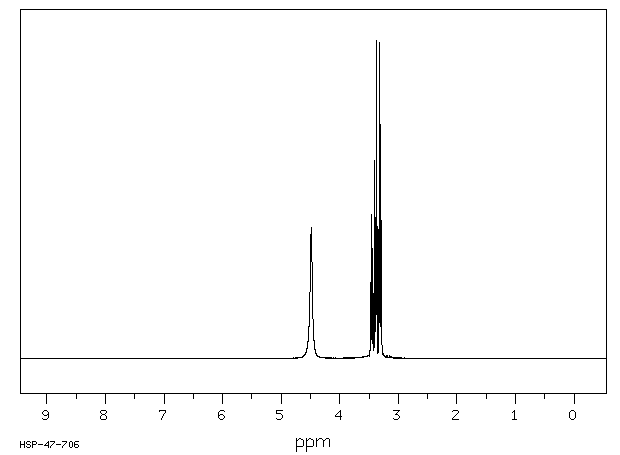# H-NMR Equivalent Protons and Coupling of Glycerol

I've been searching for explanations to understand why the labeled protons on carbons 1 and 3 are not equivalent in the below figure.Another figure that shows the same results:SDBS confirms the same, although the assignments of the signals show that all 3 hydroxyl Hs are equivalent, which I don't understand.I would think that all 4 hydrogens on C1 and C2 would be in identical environments but it seems this is incorrect. In fact, the figures show that the two hydrogens on C1 (and C2) are not equivalent to each other. Why is it that the hydrogens on the terminal carbons are not equivalent, but rather each hydrogen on one terminal carbon has an equivalent hydrogen partner on the other?

Also, what is the explanation for why SDBS shows all 3 hydroxyl protons as equivalent? Why would the -OH on C2 be equivalent to the ones on the terminal carbons?

Edit: As for my first question, I think I found the correct explanation. I believe it's because the hydrogens pairs are either diastereotopic or enantiotopic.

• They're diastereotopic as you suspect! Jun 2, 2018 at 10:55
• The couplings shown at the top are wrong. $\ce{H_c}$ to $\ce{H_a}$ is not $\pu{12 Hz},$ as it's way too big and is in fact the geminal coupling between $\ce{H_a}$ and $\ce{H_b}.$ The actual couplings are $11.7,$ $6.3$ and $\pu{4.2 Hz}$ for $\ce{H_a}/\ce{H_b},$ and $\ce{H_c}$ to $\ce{H_a}$ or $\ce{H_b},$ respectively. The hydrogens on the $\ce{OH}$ will not show up in exchanging solvents like $\ce{D2O}/\ce{H2O},$ but can in $\ce{CDCl3}.$ Mar 17, 2021 at 19:28
• See a newer answer to this question: chemistry.stackexchange.com/questions/144088/… Mar 17, 2021 at 20:49